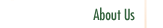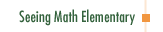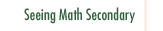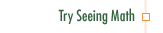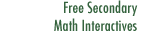Number & Operations:
The Magnitude of Fractions

In Numbers & Operations: The Magnitude of Fractions, we expand the concept of fractions. Students already understand fractions as parts of a whole. By comparing fractions, students come to understand them as something more—numbers that have magnitude. This is the central goal of the lesson.

Participants in this course:

• Examine the understanding of fractions both as parts of a whole and as quantities in their own right.
• Explore research into how students learn to understand fractions and learn new meanings of fractions beyond "part/whole."
• See how using manipulatives can either assist or impede efforts to understand and represent fractions.
• Analyze why manipulatives are sometimes ill-suited to represent certain meanings of fraction, such as fraction as a numerical quantity.
• See how students can learn to compare the magnitude of a familiar fraction (such as 1/4, 1/3, 1/2, 2/3, and 3/4) with other fractions that have different denominators.
• Examine how diagrams can help students understand fractions and build students' capacity to solve problems.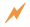Click to Get Seeing Math™ ElementaryCourse Listing Effective Questioning in the Mathematics Classroom Foundations of EffectiveMathematics Teaching Formative Assessment in the Mathematics Classroom Number & Operations: Division with Remainders Number & Operations: The Magnitude of Fractions Number & Operations: Broken Calculator Geometry: 2D and 3D Figures Geometry: Calculating Area of a Triangle Data Analysis and Probability: Using Data to Make Predictions Data Analysis and Probability: Measures of Center Pre-Algebra: Pan Balance Equations Pre-Algebra: Patterns and Functions